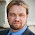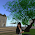Skip to main content

### Testing Android Blogger

Hopefully this works!

$y' = \frac{dy}{dx} = \frac{1}{x^2}$

### Comments

1.Works perfectly!

2.how did you ? :)

### A Bayes' Solution to Monty Hall

For any problem involving conditional probabilities one of your greatest allies is Bayes' Theorem. Bayes' Theorem says that for two events A and B, the probability of A given B is related to the probability of B given A in a specific way.

Standard notation:

probability of A given B is written $$\Pr(A \mid B)$$
probability of B is written $$\Pr(B)$$

Bayes' Theorem:

Using the notation above, Bayes' Theorem can be written: $\Pr(A \mid B) = \frac{\Pr(B \mid A)\times \Pr(A)}{\Pr(B)}$Let's apply Bayes' Theorem to the Monty Hall problem. If you recall, we're told that behind three doors there are two goats and one car, all randomly placed. We initially choose a door, and then Monty, who knows what's behind the doors, always shows us a goat behind one of the remaining doors. He can always do this as there are two goats; if we chose the car initially, Monty picks one of the two doors with a goat behind it at random.

Assume we pick Door 1 and then Monty sho…

### Mixed Models in R - Bigger, Faster, Stronger

When you start doing more advanced sports analytics you'll eventually starting working with what are known as hierarchical, nested or mixed effects models. These are models that contain both fixed and random effects. There are multiple ways of defining fixed vs random random effects, but one way I find particularly useful is that random effects are being "predicted" rather than "estimated", and this in turn involves some "shrinkage" towards the mean.

Here's some R code for NCAA ice hockey power rankings using a nested Poisson model (which can be found in my hockey GitHub repository):
model <- gs ~ year+field+d_div+o_div+game_length+(1|offense)+(1|defense)+(1|game_id) fit <- glmer(model, data=g, verbose=TRUE, family=poisson(link=log) ) The fixed effects are year, field (home/away/neutral), d_div (NCAA division of the defense), o_div (NCAA division of the offense) and game_length (number of overtime periods); off…

### Notes on Setting up a Titan V under Ubuntu 17.04

I recently purchased a Titan V GPU to use for machine and deep learning, and in the process of installing the latest Nvidia driver's hosed my Ubuntu 16.04 install. I was overdue for a fresh install of Linux, anyway, so I decided to upgrade some of my drives at the same time. Here are some of my notes for the process I went through to get the Titan V working perfectly with TensorFlow 1.5 under Ubuntu 17.04.

Old install:
Ubuntu 16.04
EVGA GeForce GTX Titan SuperClocked 6GB
2TB Seagate NAS HDD
+ additional drives

New install:
Ubuntu 17.04
Titan V 12GB
/ partition on a 250GB Samsung 840 Pro SSD (had an extra around)
/home partition on a new 1TB Crucial MX500 SSD
New WD Blue 4TB HDD
+ additional drives

You'll need to install Linux in legacy mode, not UEFI, in order to use Nvidia's proprietary drivers for the Titan V. Note that Linux will cheerfully boot in UEFI mode, but will not load any proprietary drivers (including Nvidia's). You'll need proprietary drivers for Tens…